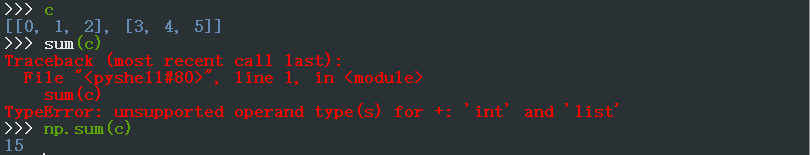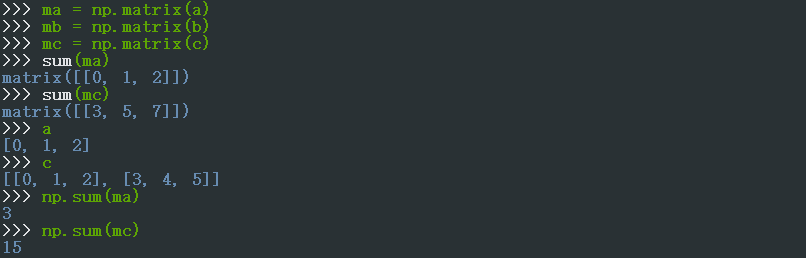# python自带的sum()函数和numpy库中的sum()函数的区别

return np.sqrt(sum(np.power(vecA - vecB, 2)))

matrix([[ 5.112672,  0.860815]])return np.sqrt(np.sum(np.power(vecA - vecB, 2)))